# Two proofs complex matrices have eigenvalues

Today I will briefly discuss two proofs that every matrixover the complex numbers (or more generally, over an algebraically closed field) has an eigenvalue. Notice that this is equivalent to finding a complex number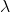such thathas nontrivial kernel. The first proof uses facts about “linear dependence” and the second uses determinants and the characteristic polynomial. The first proof is drawn from Axler’s textbook ; the second is the standard proof.

## Proof by linear dependence

Let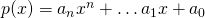be a polynomial with complex coefficients. Ifis a linear map,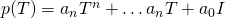. We think of this as “evaluated at”.

Exercise: Show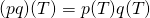.

Proof: Pick a random vector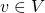. Consider the sequence of vectors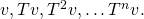This is a set of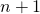vectors, so they must be linearly dependent. Thus there exist constantssuch that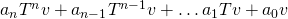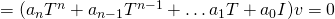.

Define. Then, we can factor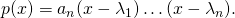By the Exercise, this implies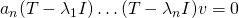. So, at least one of the maps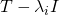has a nontrivial kernel, sohas an eigenvalue.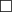## Proof by the characteristic polynomial

Proof: We want to show that there exists somesuch thathas nontrivial kernel: in other words, thatis singular. A matrix is singular if and only if its determinant is nonzero. So, let; this is a polynomial in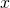, called the characteristic polynomial of. Now, every polynomial has a complex root, say. This implies, sohas an eigenvalue.## Thoughts

To me, it seems like the determinant based proof is more straightforward, although it requires more machinery. Also, the determinant based proof is “constructive”, in that we can actually find all the eigenvalues by factoring the characteristic polynomial. On subject of determinant-based vs determinant-free approaches to linear algebra, see Axler’s article “Down With Determinants!” .

There is a similar situation for the problem of showing that the sum (or product) of two algebraic numbers is algebraic. Here there is a non-constructive proof using “linear dependence” (which I attempted to describe in a previous post) and a constructive proof using the characteristic polynomial (which will hopefully be the subject of a future blog post). A further advantage of the determinant-based proof is that it can be used more generally to show that the sum and product of integral elements over a ring are integral. In this more general context, we no longer have linear dependence available.

## References

1. Sheldon Axler, Linear algebra done right. Springer 2017
2. Evan Chen, An Infinitely Large Napkin, available online
3. Sheldon Axler. Down with Determinants! The American Mathematical Monthly, 102(2), 139, 1995. doi:10.2307/2975348, available online